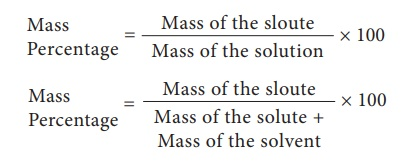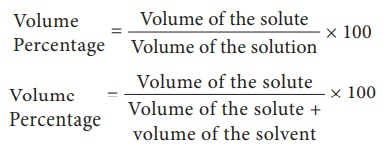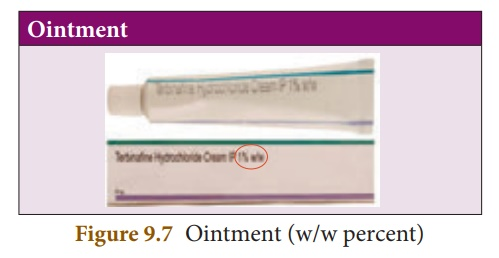Home | | Science 10th Std | Concentration of a Solution

# Concentration of a Solution

Concentration of a solution may be defined as the amount of solute present in a given amount of solution or solvent.

Concentration of a Solution

So far, we discussed what is a solution? what does it consist of and its types. Most of the chemical reactions take place in solutions form. So it is essential to quantify the solute in solvent to study the reactions. To quantify the solute in a solution, we can use the term “concentration”.

Concentration of a solution may be defined as the amount of solute present in a given amount of solution or solvent.

Quantitatively, concentration of a solution may be expressed in different methods. But here, we shall discuss percentage by mass (% mass) and percentage by volume (% volume).

## 1. Mass percentage

Mass percentage of a solution is defined as the percentage by mass of the solute present in the solution. It is mostly used when solute is solid and solvent is liquid.For example: 5% sugar solution (by mass) means 5 g of sugar in 95 g of water. Hence it is made 100g of solution.

Usually, mass percentage is expressed as w/w (weight / weight); mass percentage is independent of temperature.

## 2. Volume percentage

Volume percentage is defined as the percentage by volume of solute (in ml) present in the given volume of the solution.For example, 10% by volume of the solution of ethanol in water, means 10 ml of ethanol in 100 ml of solution (or 90 ml of water)

Usually volume percentage is expressed as v/v (volume / volume). It is used when both the solute and solvent are liquids. Volume percentage decreases with increases in temperature, because of expansion of liquid.

You can notice that in the commercial products that we come across in our daily life such as a solution of syrups, mouth wash, antiseptic solution, household disinfectants etc., the concentration of the ingredients is expressed as v/v. Similarly, in ointments, antacid, soaps, etc., the concentration of solutions are expressed as w/w.Study Material, Lecturing Notes, Assignment, Reference, Wiki description explanation, brief detail
10th Science : Chapter 9 : Solutions : Concentration of a Solution |